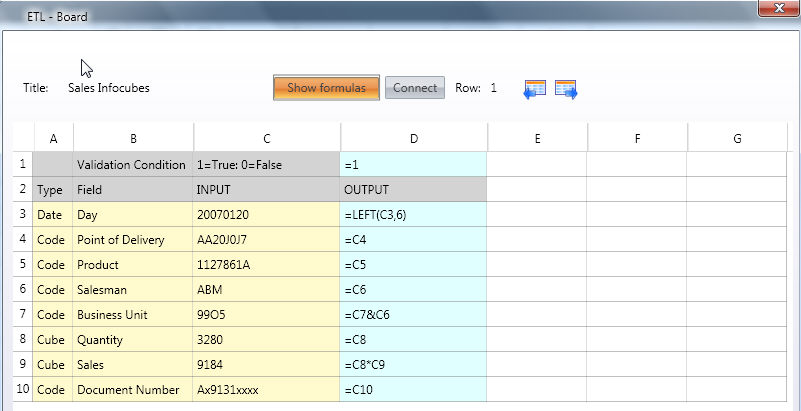﻿ Defining an ETL transformation and validation rule

# Defining an ETL transformation and validation rule

The ETL function allows you to add data transformation and validation rules to a Data Reader protocol.

The data flow of incoming data starts with retrieving data from the different data sources through a Datareader protocol, which then applies some validation and transformation formulas (the ETL rules) and then data is loaded in the board entites and cubes.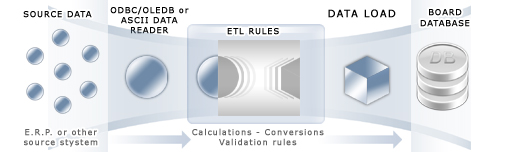The formulas and validation rules defined in the ETL are calculated record by record on the incoming data. An ETL formula can refer to any field of the record. The transformed record, i.e. the result of the calculation, is the output ETL. This output is the data which is actually loaded into the Board database.

To define an ETL set of rules on a Datareader protocol, click on the ETL icon on the protocol row. Note that it is necessary to create a protocol first, then you can configure the ETL for it.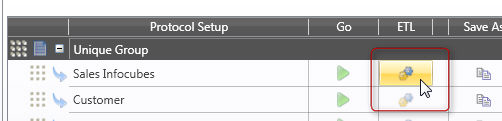The ETL definition window is a spreadsheet-like environment,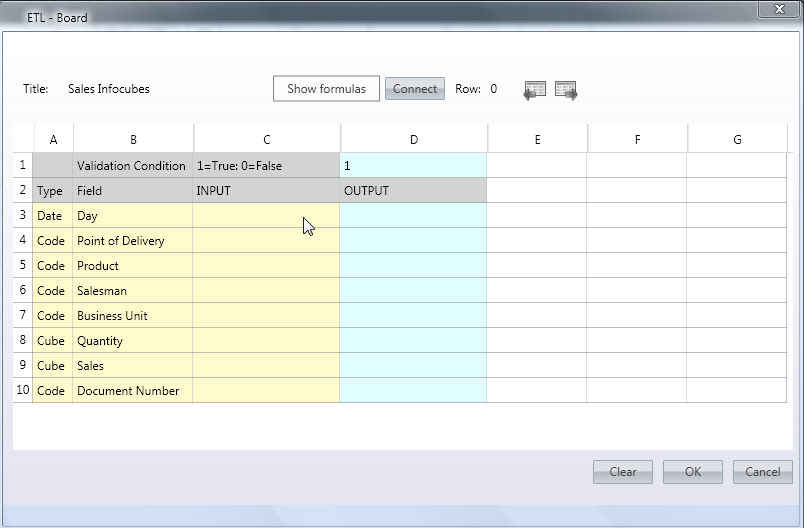The InfoCubes and Entities of the reader protocol are displayed in columns A and B (Type and Field). The ETL input (shown in column C) is the incoming external data from the source system (an ASCII file or an ODBC data source). The ETL output, shown in column D, is the transformed data (after applying the formulas) used to feed the Entities and InfoCubes.

If the ETL is associated to a text file protocol, press the Refresh file list button, then select the desired file from the drop-down list. If the ETL is associated to an OLE DB protocol, press the Connect button.

After doing so, the first record of the source file or of the source table is shown in the Input column. It is possible to scroll the first 200 records using the previous/next icons (left and right arrows)..

Click the "Show Folmulas" button, then in the Output column, D, type the desired expression using the usual syntax of the spreadsheet formulas. No transformations are defined by default therefore each cell of the Output column is equal to the equivalent cell from the Input column. Columns E to G can be used to write intermediate calculations thus simplifying the definition of long complex expressions. To revert to preview mode, click again the "Show Formulas" button.

In cell D1, it is possible to define a record validation formula. If the result of the expression is 1 (true), the record is validated and fed into Board database, if the result of the expression is 0 (False), the record is discarded. The expression can refer to any cell of columns C to G.

Example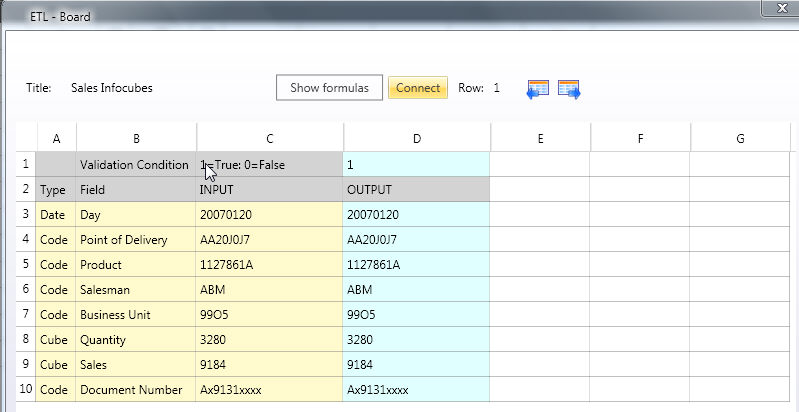The transformations defined in the above example are common spreadsheet formulas. click the Show formulas button to edit or view the formulas for each field.